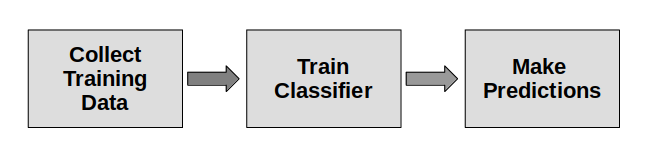### Neural Network Example

In this article we’ll make a classifier using an artificial neural network.
While internally the neural network algorithm works different from other supervised learning algorithms, the steps are the same:Related course:

## Training data

We start with training data:

 Array Contains Size X training samples represented as floating point feature vectors size (n_samples, n_features) y class labels for the training samples size (n_samples,)

In code we define that as:

## Train classifier

We then create the classifier:

Train the classifier with training data:

## Predict

And finally we can make predictions:

The neural network code is then: